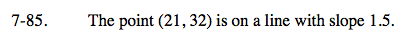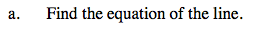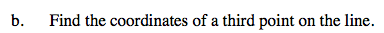### Home > AC > Chapter 7 > Lesson 7.3.1 > Problem7-85

7-85.The equation of a line is in the y = mx + b format, where m = slope (growth) and b = y-intercept (starting point).

Substitute the slope and the (x, y) point given in the problem into y = mx + b.

y = mx + b
y = 1.5x + b
32 = (1.5)(21) + b

Solve for b.

b = 0.5
y = 1.5x + 0.5

Don't forget to check your solution!One way to find this is to substitute an x or y value of your choice into the equation you found in part (a).

Sam substitutes the value x = 4 into the equation.

y = 1.5 (4) + 0.5
y = 6.5

The coordinates of the third point that Sam found are (4, 6.5).